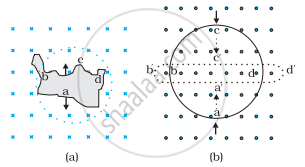# Use Lenz’s law to determine the direction of induced current in the situations described by Fig.: - Physics

Use Lenz’s law to determine the direction of induced current in the situations described by Fig.:

(a) A wire of irregular shape turning into a circular shape;

(b) A circular loop being deformed into a narrow straight wire.#### Solution

According to Lenz’s law, the direction of the induced emf is such that it tends to produce a current that opposes the change in the magnetic flux that produced it.

(a) When the shape of the wire changes, the flux piercing through the unit surface area increases. As a result, the induced current produces an opposing flux. Hence, the induced current flows along adcb.

(b) When the shape of a circular loop is deformed into a narrow straight wire, the flux piercing the surface decreases. Hence, the induced current flows along a'd'c'b'.

Concept: Lenz’s Law and Conservation of Energy
Is there an error in this question or solution?

#### APPEARS IN

NCERT Physics Part 1 and 2 Class 12
Chapter 6 Electromagnetic Induction
Exercise | Q 6.2 | Page 230
NCERT Class 12 Physics Textbook
Chapter 6 Electromagnetic Induction
Exercise | Q 2 | Page 230

Share